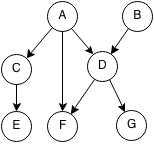## Introduction

Prerequisites: Graph Theory, Depth First Search

A topological sort or topological order of a directed graph is an order in which every node comes after its ancestors.For example topological orders could be:

• (A, B, C, D, E, F, G)
• (B, A, D, C, F, E, G)
• (B, A, D, G, F, C, E)

But (B, A, C, F, D, E, G) is not a topological ordering because D is an ancestor of F and it comes after F.

## Implementation

Topological sort can implemented in O(n) time using DFS for a directed acyclic graph (a digraph with no cycles). How it works:

2. Pick any unmarked node.
3. Get the DFS preordering from that node for unvisited nodes.
5. Mark every node that has been visited.Example:

• Pick C
• DFS preorder from C is (C,E)